# Monte Carlo simulation of colloidal membrane filtration: Model development with application to characterization of colloid phase transition, страница 3

So, colloidal membrane filtration encompasses a complex network of counteracting physical processes that calls for a microscopic modeling technique to accurately and precisely describe the system. The purpose of this study is to present a Monte Carlo simulation model of particle deposition dynamics during membrane filtration. We will focus on the issues pertinent to the fundamental algorithms and parameters for developing the model. Two of the primary forces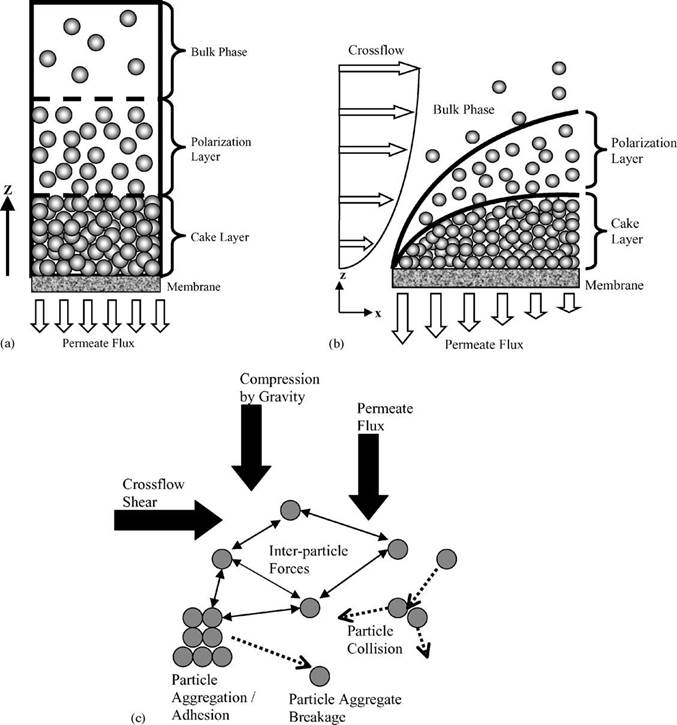Fig. 1. (a) Schematic of a dead-end filtration system, (b) schematic of a crossflow filtration system, and (c) outline of forces driving colloidal transport,

aggregation, and breakage.

shown in Fig. 1(c), the downward hydrodynamic drag and the inter-particle interactions, will be incorporated into the model. The model will then be applied to investigate the phase transition phenomenon of the polarized layer from fluid to solid. We will relate the observation of a point of critical flux during filtration to the occurrence of phase transition. Multiple possible definitions of critical flux are available and we will explore the applicability of each [16,17]. The significance of each proposed point of phase transition in relationship to membrane filtration performance will be examined.

Further extensions from the current model to include the complete set of forces as shown in Fig. 1(c) will culminate in a complete Monte Carlo simulation model that remains true to the actual physical situation and can effectively investigate significant issues such as phase transition during crossflow filtration, among others.

## 2.2.  Governing equations for the system

### 2.2.1.  Equations for mass transport

The governing equation for colloidal transport in a deadend filtration system is [5,18]: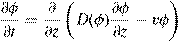(1)

with boundary condition:

φ = φb at t = 0,                  φ = φb at z = δcp,

∂φ

D(φm)= vφm at z = 0                                                    (2)

∂z

where φ is the volume fraction of particles, φb the bulk volume fraction, φm the particle volume fraction on the membrane surface, D(φ) the concentration-dependent diffusion coefficient of particles , t the time, z the axis perpendicular to the membrane surface, δcp the thickness of the polarization layer, and v the permeate flux.

Likewise for a crossflow system the governing equation for mass transport becomes [5,18]: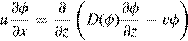(3)

with boundary conditions:

φ = φb at z = δcp,                  φ = φb at x = 0,

∂φ

D(φm)= vφm at z = 0                                                    (4)

∂z

where u is the additional crossflow velocity in the axial, x, direction. It should be noted from a comparison of Eqs. (1) and (3) that dead-end filtration is inherently a time-dependent, transient process while crossflow filtration will reach a timeindependent steady state of operation. The boundary condition given in Eq. (4) applies for the case of the polarization layer in the absence of cake formation.

### 2.2.2.  Equations for filtration

Ingeneral,oneofthequantitiesofspecialinterestinapplications of membrane filtration is the permeate flux which, for both dead-end and crossflow filtration (after cake formation has occurred), is governed by Darcy’s Law :

vw =−v =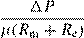(5)

where vw is the permeate flux in magnitude, P the applied pressure within the system, µ the absolute fluid viscosity, Rm the membrane resistance, and Rc the cake layer resistance further defined as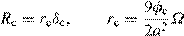(6)

where rc is the specific cake resistance, δc the cake layer thickness, a the particle radius, φc the cake layer volume fraction and Ω the Happel’s correction factor defined as :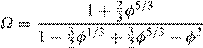(7)

The model presented in this study will utilize MC simulation to compute the volume fraction to be inputted into Eqs. (1)–(7) under given operating conditions to obtain the system response.